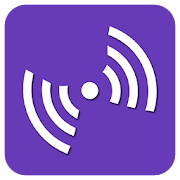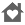Acoustics Engineering PackEveryone
11
Acoustics Engineering Pack contains 94 Calculators and References, that can quickly and easily calculate and helps you to refer different Acoustical Engineering parameters. Automatic & Accurate Calculations and Conversions with every Unit and Value Changes. A Complete Acoustical Engineering Dictionary.

* Available in English, Français, Español, Italiano, Deutsch, Português & Nederlands *

Acoustics Engineering Pack contains following 94 Calculators and References:
• Absorption Coefficients of Building Materials and Finishes
• Acceleration Converter
• Acoustic Flow Meter
• Acoustic Impedance
• Acoustic Intensity and Sound Intensity Level Relationship
• Acoustic Power and Sound Power Level Relationship
• Area Converter
• Bragg's Law
• Capacitive Reactance
• Coincidence
• Cutoff Frequency
• Damping Factor
• Data Transfer Converter
• Day Night Sound Level
• Density Converter
• Diffraction Grating Equation
• Doppler Effect - Approaching Receiver
• Doppler Effect - Approaching Source
• Doppler Effect - Receding Receiver
• Doppler Effect - Receding Source
• Doppler Effect - Wavelength Behind
• Doppler Effect - Wavelength Front
• Electrical Harmonics
• Energy Converter
• Force Converter
• Frequency Converter
• Frequency Limits (1/3-Octave bands)
• Frequency Limits (Octave bands)
• Fresnel Number
• Inductive Reactance
• Inverse Square Law
• Length Converter
• Level Damping
• Mach Number
• Magnetic Flux Converter
• Magnetic Flux Level
• Mass Converter
• Mean Absorption Coefficient
• Metric Weight Converter
• Noise Criterion (Location)
• Noise Criterion (Sound Pressure Levels)
• Noise Exposure Level
• Noise Exposure Level - Duration
• Noise Generation - Ducts
• Noise Pollution Level
• Noise Rating Curves
• Ohm's Law of Acoustics (Acoustic Impedance)
• Ohm's Law of Acoustics (Particle Velocity)
• Ohm's Law of Acoustics (Sound Intensity)
• Ohm's Law of Acoustics (Sound Pressure)
• Outdoor Ambient Sound Levels
• Particle Velocity and Particle Velocity Level Relationship
• Power Converter
• Preferred Noise Criterion
• Prefixes Converter
• Pressure Converter
• Quality Factor
• Resonant Frequency
• Reverberation Time
• RMS Noise
• Room Constant
• Room Criteria
• Sound Absorption Coefficient
• Sound Absorption Coefficient (Common Materials)
• Sound Attenuation
• Sound Attenuation Level - Main Duct to Branches
• Sound Converter
• Sound Energy and Sound Energy Level Relationship
• Sound Energy Density and Sound Density Level Relationship
• Sound Intensity Level
• Sound Power Emitted
• Sound Power Level
• Sound Pressure - Recommended Maximum Levels in Rooms
• Sound Pressure and Sound Pressure Level Relationship
• Sound Pressure Level
• Sound Pressure Level (Linear Sound Source)
• Sound Speed
• Sound Transmission Loss - Building Elements
• Sound Transmission through Duct Walls
• Sound Wavelength
• Speed of Sound (Common Liquids)
• Speed of Sound (Common Solids)
• Speed of Sound (Gases)
• Temperature Converter
• Time Converter
• Velocity Converter
• Voltage to Voltage Level Conversion
• Volume Converter
• Wave Frequency
• Wave Velocity

Key Features:
• Complete coverage of calculators and references in Acoustic Engineering field.
• Professionally and Newly designed user interface that speeds up Data Entry, Easy Viewing and Calculation Speed.
• Automatic Calculation of the output with respect to changes in the Input, Options and Units.
• Formulas are provided for each calculator.
• Extremely Accurate Calculators and Pleasant Presentation of References.

Complete Acoustical Engineering Guide
Collapse

Review Policy
3.5
11 total
5
4
3
2
1

What's New

Acoustics Engineering Pack
Collapse

Eligible for Family LibraryLearn More
Updated
May 7, 2018
Size
1.5M
Installs
500+
Current Version
5.0
Requires Android
4.0 and up
Content Rating
Everyone
Permissions
Offered By
Sparkle Solutions
Developer
Sparkle Solutions, New No.13, Old No.26, 4th Street, SBI Staff Colony, Arumbakkam, Chennai - 600106, TamilNadu, India.